# AFCAT Exam Topic Based Important Sample Question Answer Set 1

In this article we are sharing AFCAT Exam Topic Based Important Sample Question Answer Set 1, soon we will publish set 2.## AFCAT Exam Topic Based Important Sample Question Answer Set 1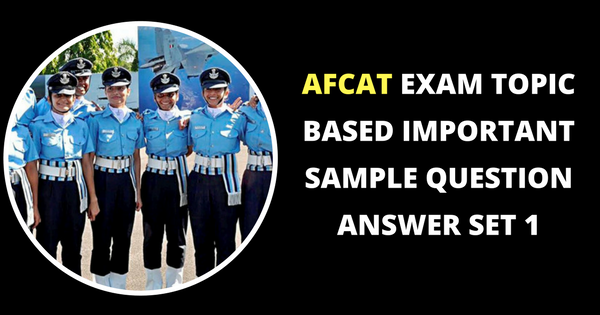Q.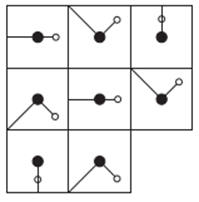Which is the missing tile?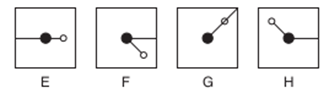a. E b. F c. G d. H Solution. E: looking across, the long line is moving 45° clockwise at each stage and the small line 45° anti clockwise. Looking down, the reverse is happening. Answer. a
 Q.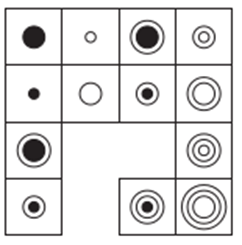Which section is missing?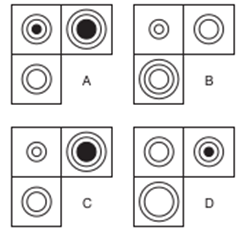a. A b. B c. C d. D Solution. C: looking across and down, a circle is added to each alternate square Answer. c
 Q. Directions — In these tests you will find an Incomplete Figure and four Answer Figures. You have to select one diagram from the Answer Figures which fits into the blank column in Incomplete Figure in order to complete it: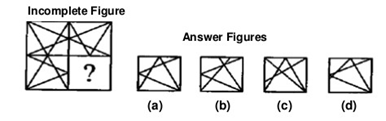a. (a) b. (b) c. (c) d. (d) Solution. Create a mirror image of the bottom left box and compare with the options for a match Answer. a
 Q.  Directions — In these tests find which code matches the shape or pattern given at the end of each question.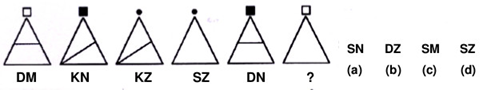a. (a) b. (b) c. (c) d. (d) Solution. The figure at the end is made by combining the structural patterns of DM and SZ figures, new figure is DZ. Answer. b
 Q. complete the figure : In each of the following questions, select a figure from amongst the four alternatives, which when placed in the blank space of figure (X) would complete the pattern. 10.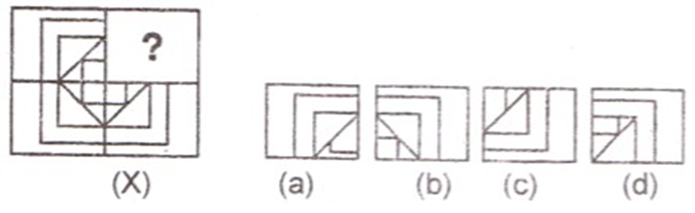a. (a) b. (b) c. (c) d. (d) Answer. b
 Q. The simple interest accrued on a sum of money at the end of four years is 1 /5th of its principal. What is the rate of interest per annum? a. 4% b. 5% c. 6% d. Inadequate data Solution. Use s = (PRT) / 100 Answer. b
 Q. Calculate the amount on 1875 for 2 years at 4% per annum, compounded yearly. a. 676 b. 776 c. 1778 d. 2028 Solution.  apply CI formula for 2 yrs: 1875 (1 + 4/100)^2 = 2028 Answer. d
 Q. A boy was asked to multiply a number by 25. Instead, he multiplied the number by 52 and got the answer 324 more than the correct answer. The number to be multiplied was a. 12 b. 15 c. 25 d. 32 Solution.Answer. a
 Q. Out of the 1000 inhabitants of a town, 60% are male of whom 20% are literate. If, amongst all the inhabitants, 25% are literate, then what percentages of the females of the town are literate? a. 22.5 b. 32.5 c. 27.5 d. 37.5 Solution.Answer. b
 Q. Rs.800 becomes Rs.956 in 3 years at a certain rate of interest. If the rate of interest is increased by 4% what amount will Rs.800 become in 3 years? a. Rs 1020 b. Rs 1052 c. Rs 1282 d. Rs 1080 Solution.  S.I. = 956 – 800 = Rs 156 R = 156×100/800×3 R = 6(1/2) + 4 =21/2 SI = 800  x 21/2 x 3100 = 252 Now amount will be 800 + 252 = 1052 S.I. = 800×212×3100=252S.I. = 800×212×3100=252 Answer. b
 Q. A man has Rs 640 in the denomination of one rupee, five rupee and ten rupee notes. The numbers of each type of notes are equal. What is the total number of notes he has? a. 60 b. 90 c. 150 d. 120 Solution. Let ‘x’ be the number of each type of note. x + 5x + 10x = 640 16x = 640 X = 40 Therefore the man has 40 one rupee note, 40 five rupee note and 40 ten rupee nope and in total 120. Answer. d
 Q. A mixture of 40 liters of milk and water contains 10% water. How much water should be added to this mixture so that the new mixture contains 20% water? a. 4 liters b. 5 liters c. 6.5 liters d. 7.5 liters Solution. Milk = 90/(100) x 40 = 36 litres, water = 4 litres. Let x litres of water be added. Then (x + 4))(40 + x) x 100 = 1/5 :. 5x + 20 = 40 + x => x = 5.` Answer. b
 18. Reena took a loan of Rs 1200 with simple interest for a certain number of years. The number of years is same as the interest rate. If she has paid Rs 432 as interest at the end of the loan period, what was the rate of interest? a. 3.6 b. 6 c. 12 d. None of these Solution.Answer. b
 Q. Find the average of all the numbers between 6 and 34 which are divisible by five. a. 18 b. 20 c. 20 d. 30 Solution.Answer. b
 Q. If the selling price of an article is 4/3 of its cost price, the profit in transaction is a. 16.75% b. 20.50% c. 25.50% d. 33.33% Solution. Lets assume CP as Rs.300/- So SP will be 300 x (4/3) = Rs.400/- Profit = SP-CP= Rs.100/- ie if SP is 4/3=(1.3333) then CP will be 1 and profit will be 1.33333–1=0.3333 1/3 = 0.3333 Answer. d